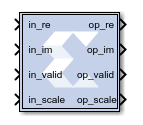# Vector FFT - 2020.2 English

## Vivado Design Suite Reference Guide: Model-Based DSP Design Using System Generator (UG958)

Document ID
UG958
Release Date
2020-11-18
Version
2020.2 English

The Vector FFT block supports the FFT operation for vector type inputs.

## Description

The real part of the input data should be given to the `in_re` port, and the imaginary part should be given to the `in_im port`.

When the `in_valid` is high it indicates that the input data is valid. When `out_valid` is high, it indicates that the output data is valid. The `in_scale` input port is used if scaling is required. And `out_scale` is used in if there is an internal overflow.## Data Type Support

• The number of `in_scale` bits must be equal to `log2(FFT length)`.
• `in_valid` and `out_valid` are of Boolean data type.

## Block Parameters

FFT length (N) is the size of the transformation, and should be powers of 2 in the range of 2^3 to 2^16. SSR is the super sample rate, the number of samples processed in parallel every clock. Using a typical example with N=1024 and SSR=4, the core would compute one 1K FFT every 256 clock cycles, processing 4 input samples/clock.

The fixed-point output data size must be 27 bits or less, this is limited by the DSP48 multiplier A port size.

BRAM_THRESHOLD is an implementation parameter with no functional implications, it controls the use of distributed RAM vs BRAM when implementing delay lines. It can be used to trade utilization numbers between these two types of resources. The higher the value, the more distributed RAM will be used instead of BRAM. Typical values to try are 258, 514, and 1026.

Scaling Ports

The scaling ports are called SI and SO. Their width matches the FFT size N, it is always log2(N). There is one SI bit for every add/subtract stage, where internal overflows can occur. If that bit is set to zero then no scaling happens and bit growth is addressed by increasing the internal data sizes bit, one bit every stage. If the bit is set to 1 then the stage divides by 2, and no internal data growth is required to prevent overflows.

Generally, if the output data size is log2(N) bits larger than the input size, no scaling is required, and SI is set to all zeros. If the input and output data sizes are equal, then scaling on every stage is needed and SI should be set to all ones. In reality, scaling is data dependent and some combination of output size growth and non-zero SI bits are used. If partial scaling is used, the non-zero bits of SI should be the MSB ones. SI should be static, it should not change while data is being processed by the core (when VI is high).

Another important requirement to avoid internal overflows is to have one MSB margin bit at the data inputs, that is the two MSBs of I.RE and I.IM should be the same. This prevents overflows ink complex multipliers. If the two rules outlined above are followed, then internal overflows are impossible by design.

The SO port is an indicator of internal overflows, it is not normally used, only attach an unsigned signals of size log2(N) to it.